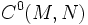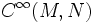# Smooth approximation theorem for differential manifolds

Let$M$ and$N$ be differential manifolds and define$C^0(M,N)$ to be the space of all continuous maps from$M$ to$N$, endowed with the compact-open topology. Let$C^\infty(M,N)$ be the space of all smooth maps from$M$ to$N$, viewed as a subset of$C^0(M,N)$. Then$C^\infty(M,N)$ is dense in$C^0(M,N)$.